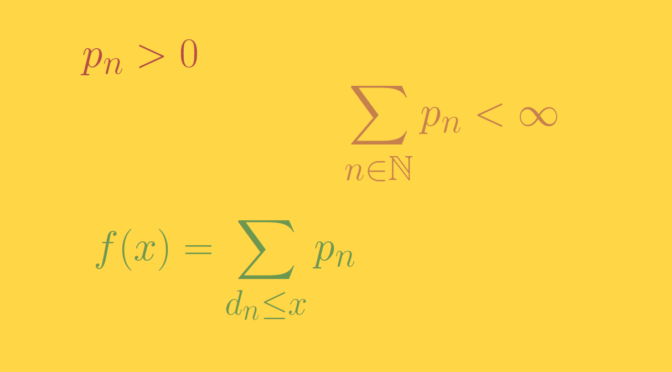# A monotonic function whose points of discontinuity form a dense set

Consider a compact interval $$[a,b] \subset \mathbb R$$ with $$a \lt b$$. Let’s build an increasing function $$f : [a,b] \to \mathbb R$$ whose points of discontinuity is an arbitrary dense subset $$D = \{d_n \ ; \ n \in \mathbb N\}$$ of $$[a,b]$$, for example $$D = \mathbb Q \cap [a,b]$$.

Let $$\sum p_n$$ be a convergent series of positive numbers whose sum is equal to $$p$$ and define $$\displaystyle f(x) = \sum_{d_n \le x} p_n$$.

### $$f$$ is strictly increasing

For $$a \le x \lt y \le b$$ we have $f(y) – f(x) = \sum_{x \lt d_n \le y} p_n \gt 0$ as the $$p_n$$ are positive and dense so it exists $$p_m \in (x, y]$$.

### $$f$$ is right-continuous on $$[a,b]$$

We pick-up $$x \in [a,b]$$. For any $$\epsilon \gt 0$$ is exists $$N \in \mathbb N$$ such that $$0 \lt \sum_{n \gt N} p_n \lt \epsilon$$. Let $$\delta > 0$$ be so small that the interval $$(x,x+\delta)$$ doesn’t contain any point in the finite set $$\{p_1, \dots, p_N\}$$. Then $0 \lt f(y) – f(x) \le \sum_{n \gt N} p_n \lt \epsilon,$ for any $$y \in (x,x+\delta)$$ proving the right-continuity of $$f$$ at $$x$$.

### $$f$$ is discontinuous at any $$x \in D$$

Suppose that $$x=d_m \in D$$. We have for $$a \le y \lt x$$ $f(x) -f(y) = \sum_{y \lt d_n \le x} p_n \gt p_m,$ proving that $$f$$ is not left-continuous at $$x$$.

### $$f$$ is continuous at all $$x \in [a,b] \setminus D$$

We only have to prove that $$f$$ is left-continuous at $$x$$. Let $$\mathbb N (x) = \{n \in \mathbb N \ ; \ d_n \lt x\}$$. Then $$f(x) = \sum_{n \in \mathbb N(x)} p_n$$ as $$x \notin D$$. For an arbitrary $$\epsilon \gt 0$$, it exists a finite subset $$\mathbb N_0 \subset \mathbb N(x)$$ such that $$\sum_{n \in \mathbb N_0} p_n \gt f(x) – \epsilon$$. For $$\delta > 0$$ such that the interval $$(x-\delta,x)$$ doesn’t contain any point of $$\mathbb N_0$$ we have for $$y \in (x-\delta,x)$$ $f(x) – \epsilon \le f(y) = \sum_{d_n \le y} p_n \lt f(x)$ as $$\mathbb N_0 \subset \{n \in \mathbb N \ ; \ d_n \le y\} \subset \mathbb N(x)$$. This allows to conclude that $$f$$ is continuous at $$x$$.